Go back to  'SOLVED EXAMPLES'

Example- 5

Find the set of real values of the parameter c so that \begin{align}&\frac{{{x^2} + 2x + c}}{{{x^2} + 4x + 3c}}\end{align} can take all real values for  $$x\in \mathbb{R}.$$

Solution: We want the given expression to assume all real values (for appropriate x), i.e, we want the range of the given expression to be  $$\mathbb{R}.$$

\begin{align}&\qquad\qquad \frac{{{x^2} + 2x + c}}{{{x^2} + 4x + 3c}} = y\\\\\ \;\;\;\;&\Rightarrow\quad \left( {1 - y} \right){x^2} + \left( {2 - 4y} \right)x + c\left( {1 - 3y} \right) = 0.\end{align}

For x to be real, the D for this equation should be non-negative

$\begin{array}{l} \Rightarrow 4{\left( {1 - 2y} \right)^2} \ge 4c\left( {1 - y} \right)\left( {1 - 3y} \right)\\\\ \Rightarrow 4{y^2} - 4y + 1 \ge c\left( {3{y^2} - 4y + 1} \right)\\\\ \Rightarrow \left( {4 - 3c} \right){y^2} + \left( {4c - 4} \right)y + \left( {1 - c} \right) \ge 0 \ldots (i)\end{array}$

Now comes the crucial step. Since we want the range of y to be $$\mathbb{R}.$$ , the constraint (i) should be satisfied by each real value of y. This means that the parabola for the left-hand side of (i) should not go below the axis for any value of y.

\begin{align}& \Rightarrow\quad \,{\rm{The \,\,discriminant \,\,for \,\,the\,\, left\,\, hand \,\,side \,\,of }}\left( {\,\,\rm{i}} \right){\rm{ \,\,cannot\,\, be\,\, positive}}\\\\ &\Rightarrow\quad D\,of{\rm{ }}\left( i \right) \le \,0\\ &\Rightarrow\quad 16{\left( {1 - c} \right)^2} \le 4\left( {1 - c} \right)\left( {4 - 3c} \right)\\\\ &\Rightarrow\quad 4{c^2} - 8c + 4 \le 3{c^2} - 7c + 4\\\\& \Rightarrow\quad {c^2} - c \le 0\\\\ &\Rightarrow\quad c\left( {c - 1} \right) \le 0\\\\ &\Rightarrow\quad 0 \le c \le 1\end{align}

You are urged to read this example carefully more than once until you understand it completely.

Example- 6

Find the minimum value of \begin{align}&f\left( x \right) = \frac{{\left( {x + a} \right)\left( {x + b} \right)}}{{\left( {x + c} \right)}}\end{align} where $$x > - \,c,\,\,\,a > c,\,\,\,b > c$$

Solution: Following the approach of the last two examples, we let

\begin{align}&\qquad\qquad y = \frac{{\left( {x + a} \right)\left( {x + b} \right)}}{{\left( {x + c} \right)}}\\ &\Rightarrow\quad {x^2} + \left( {a + b - y} \right)x + \left( {ab - cy} \right) = 0.\end{align}

Since x is real, $$D \ge 0$$

$\begin{array}{l} \Rightarrow {\left( {a + b - y} \right)^2} - 4\left( {ab - cy} \right) \ge 0\\\\ \Rightarrow {y^2} - 2\left( {a + b - 2c} \right)y + {\left( {a + b} \right)^2} - 4ab \ge 0\\\\ \Rightarrow {y^2} - 2\left( {a + b - 2c} \right)y + {\left( {a - b} \right)^2} \ge 0 \ldots (i)\end{array}$

The roots of this quadratic expression in y are

\begin{align}&{y_1},{y_2} = \frac{{2\left( {a + b - 2c} \right) \pm \sqrt {4{{\left( {a + b - 2c} \right)}^2} - 4{{\left( {a - b} \right)}^2}} }}{2}\\\,\,\,\,\,\,\,\,\,\,\,\, &\qquad\;= \left( {a + b - 2c} \right) \pm \sqrt {4ab + 4{c^2} - 4c\left( {a + b} \right)} \\\,\,\,\,\,\,\,\,\,\,\,\, &\qquad\;= a + b - 2c \pm 2\sqrt {ab + {c^2} - c\left( {a + b} \right)} \\\,\,\,\,\,\,\,\,\,\,\, &\qquad\;= a + b - 2c \pm 2\sqrt {\left( {a - c} \right)\left( {b - c} \right)} \\\,\,\,\,\,\,\,\,\,\,\, &\qquad\;= \left( {a - c} \right) + \left( {b - c} \right) \pm 2\sqrt {\left( {a - c} \right)\left( {b - c} \right)} \\\,\,\,\,\,\,\,\,\,\, &\qquad\;= {\left( {\sqrt {a - c} \pm \sqrt {b - c} } \right)^2}\end{align}

Let $${y_1} = {\left( {\sqrt {a - c} - \sqrt {b - c} } \right)^2}{\rm{and}}\,{y_2} = {\left( {\sqrt {a - c} + \sqrt {b - c} } \right)^2}$$

The solution for y in (i) is

$$y \le {y_1}\,{\rm{and }}y \ge {y_2}$$

We can discard the solution $$y \le {y_1}$$ (why ?) so that $$y \ge {y_2}.$$ The minimum value of y is y 2 or

${y_{\min }} = {\left( {\sqrt {a - c} + \sqrt {b - c} } \right)^2}$

Example- 7

Find the values of a for which the inequality $${x^2} + \left| {x - a} \right| - 3 < 0$$ is satisfied by at least one negative x.

Solution: Although this example can be solved analytically, we will follow a graphical approach here and the reader will see just how powerful thinking in terms of graphs is. The given inequality can be written as:

$\left| {x - a} \right| < 3 - {x^2}$

This should be satisfied for at least one negative value of x. Equivalently stated, the graph of $$\left| {x - a} \right|$$ should lie beneath the graph of $$\left( {3 - {x^2}} \right)$$ for at least one negative value of x.

The graph of $$3 - {x^2}$$ is fixed: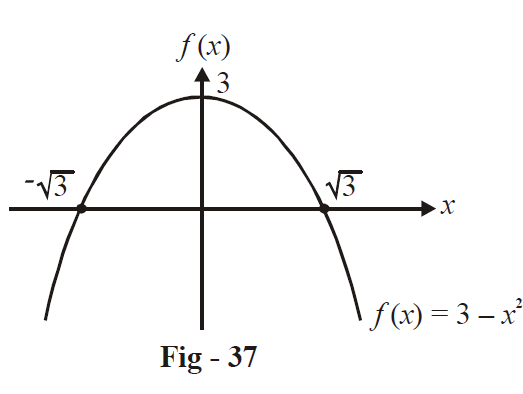The graph of $$\left| {x - a} \right|$$ is a left or right-shifted version of the graph of $$\left| x \right|$$ , depending on the value of a.

We need a to be such a value so that the graph of $$\left| {x - a} \right|$$ goes beneath that of $$\left( {3 - {x^2}} \right)$$ for some negative x.

Imagine that we start with large negative values of a so that the graph is left shifted to a large extent.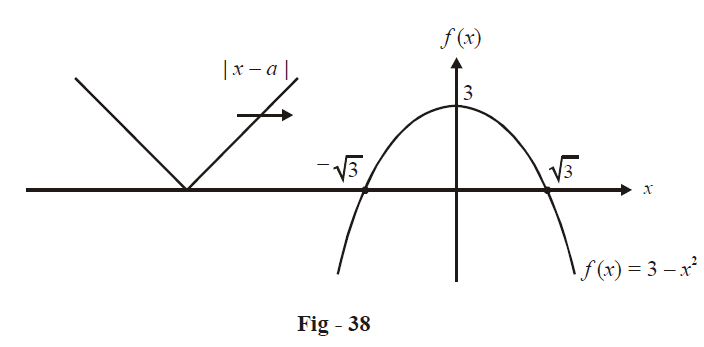As we decrease the magnitude of a, the mod-graph shifts to the right. At some point (some particular value of a, a = $$a{{~}_{1}}$$), the right arm of the mod-graph just touches the parabola (becomes a tangent to it)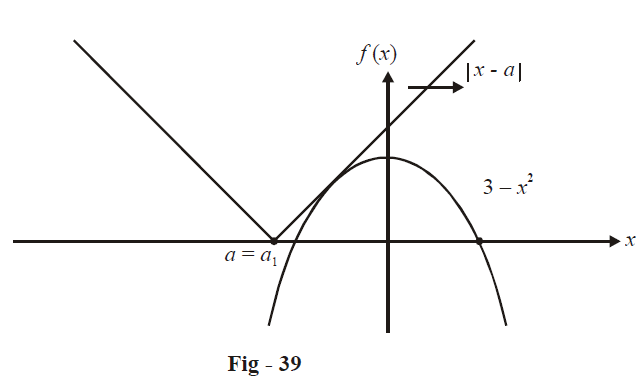As we further right-shift the mod-graph, the right arm of the mod graph goes below the parabola for some negative values of x.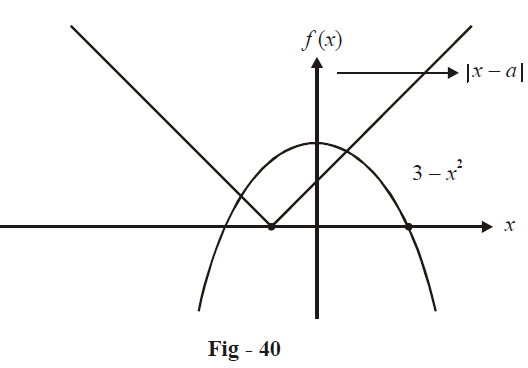In fact, after a point, the left arm also goes below the parabola for certain negative values of x (you are urged to visualise all this). The left arm remains below the parabola for certain negative x values until the other extreme is achieved (at another value of a, a =$$a{{~}_{2}}$$, when the left arm passes through the vertex of the parabola)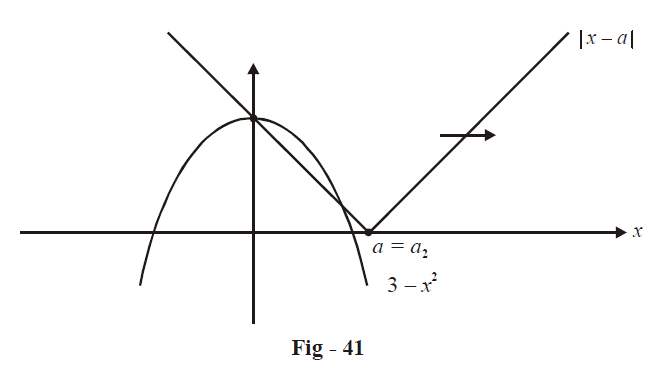Hence, when $${a_1} < a < {a_2},$$ at least one of the arms of the mod-graph will be below the parabola for some negative values of x. We can, using coordinate geometry, evaluate $$a{{~}_{1}}$$ and $$a{{~}_{2}}$$ to be - \begin{align}\frac{{13}}{4}\end{align} and 3 respectively. Therefore

$a \in \left( { - \frac{{13}}{4},3} \right)$

The description above is extremely long but you must realise that this elaborate discussion is for the sake of your understanding; while actually solving this problem in an exam all this discussion would have to be done purely in the mind!

Example- 8

For what values of the parameter a does the equation $${x^4} + 2a{x^3} + {x^2} + 2ax + 1 = 0$$ have at least two distinct negative roots.

Solution: This is a fourth degree polynomial.

Notice that the coefficients are “symmetric about the middle”, so that some useful manipulation can be done:

We divide the equation by $${{x}^{2}}$$ to get

\begin{align}{} &{x^2} + 2ax + 1 + \frac{{2a}}{x} + \frac{1}{{{x^2}}} = 0\\ &\Rightarrow {x^2} + \frac{1}{{{x^2}}} + 2a\left( {x + \frac{1}{x}} \right) + 1 = 0\\ &\Rightarrow {\left( {x + \frac{1}{x}} \right)^2} - 2 + 2a\left( {x + \frac{1}{x}} \right) + 1 = 0\\ &\Rightarrow {y^2} + 2y - 1 = 0\,\,\,\,{\rm{Where\,\, }}y = x + \frac{1}{x}\end{align}

The roots for this equation are:

$y = - a \pm \sqrt {1 + {a^2}}$

Let

\begin{align}& x + \frac{1}{x} = - a + \sqrt {1 + {a^2}} \ldots (i)\\ &\Rightarrow {x^2} + \left( {a - \sqrt {1 + {a^2}} } \right)x + 1 = 0\\ &\Rightarrow S = \sqrt {1 + {a^2}} - a > 0\\ &\quad\; P = {\rm{ }}1{\rm{ }} > {\rm{ }}0\end{align}

so that both roots of (i) are positive

Therefore, for at least two negative roots, both roots of

$x + \frac{1}{x} = - a - \sqrt {1 + {a^2}} \ldots (ii)$

should be negative

$\begin{array}{l} \Rightarrow {x^2} &+ \left( {a + \sqrt {1 + {a^2}} } \right)x + 1 = 0 \ldots (iii)\\S=& - \left( {a + \sqrt {1 + {a^2}} } \right) < 0\\P =& 1 > 0\end{array}$

Hence, both the roots will be negative. But we have to still ensure that a satisfies the basic constraint D > 0 for equation (iii)

\begin{align}&D > 0 \qquad\qquad\Rightarrow {\left( {a + \sqrt {1 + {a^2}} } \right)^2} > 4\\ &\qquad\;\;\; \qquad\qquad\Rightarrow \left( {a + \sqrt {1 + {a^2}} + 2} \right)\left( {a + \sqrt {1 + {a^2}} - 2} \right) > 0\end{align}

Since the first term is always positive, the inequality above reduces to

$\sqrt {1 + {a^2}} > 2 - a$

This can easily be solved graphically (or analytically) to get

$a > \frac{3}{4}$

Learn from the best math teachers and top your exams

• Live one on one classroom and doubt clearing
• Practice worksheets in and after class for conceptual clarity
• Personalized curriculum to keep up with school